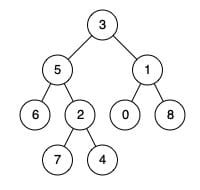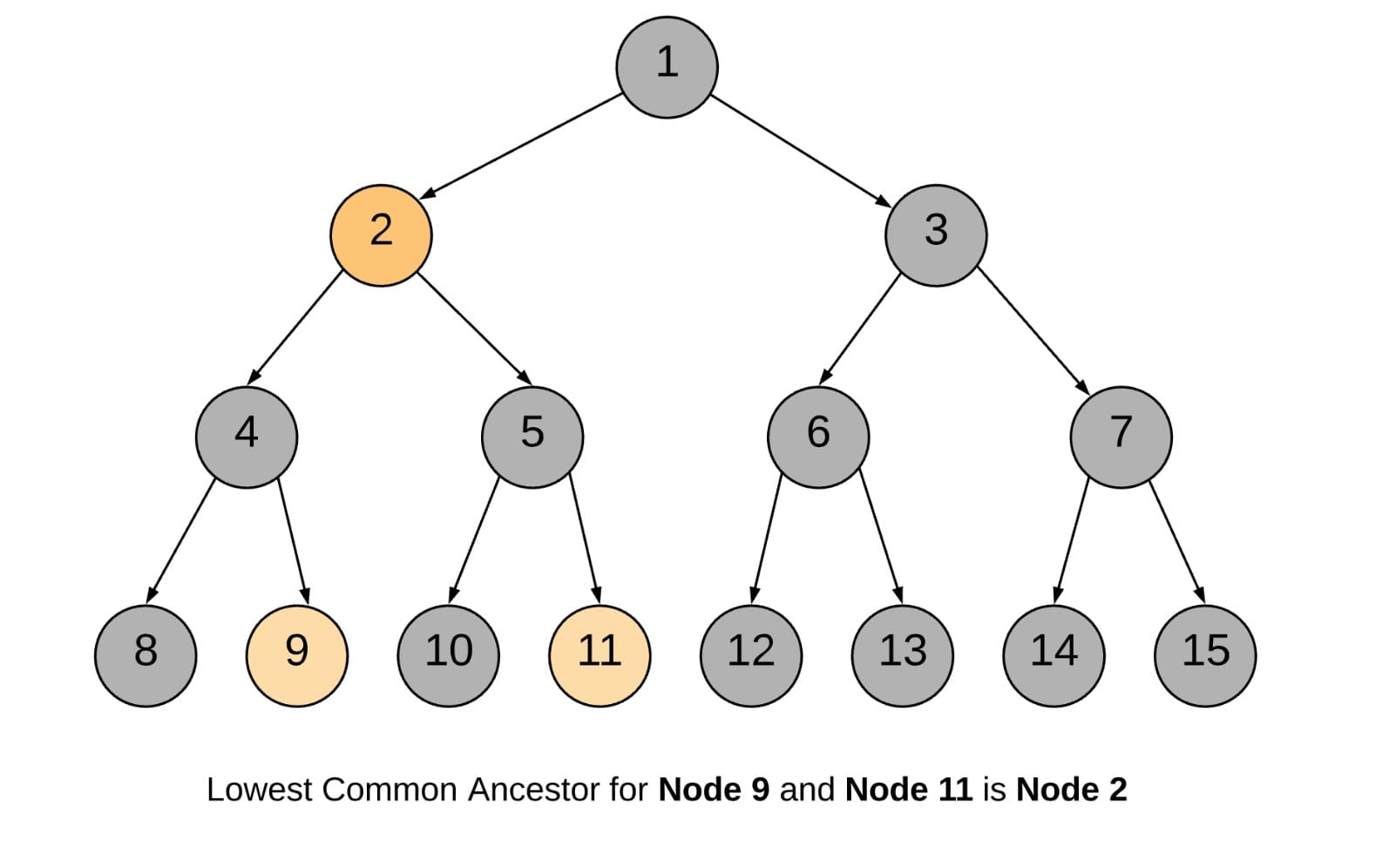# 236. Lowest Common Ancestor of a Binary Tree

Reference: LeetCode EPI 9.3EPI 9.4
Difficulty: Medium

My Post: Summary of Four Java Solutions

## Problem

Given a binary tree, find the lowest common ancestor (LCA) of two given nodes in the tree. The lowest common ancestor is defined between two nodes p and q as the lowest node in T that has both p and q as descendants (where we allow a node to be a descendant of itself).

Note:

• All of the nodes’ values will be unique.
• p and q are different and both values will exist in the binary tree.

Example:## Analysis

Methods:

1. Recursion (Brute-Force)
• Traverse the tree in a depth first manner (postorder). For a tree root, we first go deep into its left and right subtree. Remember you should believe that they will return the LCA node.
• Since the tree is unique, we don’t need to worry that both left and right calls return an LCA node. So if L or R is not null, just return it immediately.
• Also, it is a postorder traversal that explore the deepest layer first, so we don’t worry if the returned node is lowest common.
• If both L and R are null, we should check if the root is the possible LCA by using containsNode() method. It indeed incurs some repeated calculation.

Time: $O(N\log{N})$ or $O(N^2)$
Space: $O(h)$

1. Recursion (Flag)
• Traverse the tree in a depth first manner (postorder).
• If the current node itself is one of p or q, we would mark a variable mid as true and continue the search for the other node in the left and right branches.
• The LCA node would then be the node for which both the subtree recursions return a true flag, or it can also be the node which itself is one of p or q.
• If either of the left or the right branch returns true, this means one of the two nodes was found below.
• If at any point in the traversal, any two of the three flags left, right, or mid become true, this means that we have found the LCA.
• Note: We don’t return int here because it may accumulate the result making always the root the LCA.Time: $O(N)$
Space: $O(h)$

1. Iteration (Child-Parent Mapping)
• Start from the root node and traverse the tree.
• Until we find p and q both, keep storing the child-parent mappings in a hash map.
• Once we have found both p and q, we need to store all ancestors of p in a hash set pSet.
• Then, we traverse through the ancestors of q. If the ancestor is present pSet, this means is the first ancestor common between p and q, which is the LCA node.

Time: $O(N)$
Space: $O(h)$ consider the set and the map we use, but their sizes are bounded by $h$.

1. Iteration (Parent Pointers, from EPI 9.4)
• If two nodes are at the same depth, we can move up the tree in tandem from both nodes, stopping at the first node they meet.
• However, if they are not the same depth, we first ascend the deeper node and make it has the same depth with the other node. Then promote them in tandem.

Time: $O(h)$
Space: $O(1)$

CommentJunhao Wang
a software engineering cat